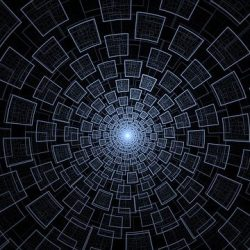# Primes Number | Primality test | Numerando.it

Photo credit:
Barbara A Lane da PixabayOn this page you can find informations about a particular class of natural numbers: Prime Numbers. You can also download tables of prime numbers less than 10.000.000 and the table of the factorization for non-prime numbers less than 500.000.

Prime numbers are positive natural numbers divisible only by 1 and themselves. These numbers represent a very particular set of natural numbers, since any natural number can be expressed as a product of prime numbers and then, in other words, we can say that if we have 'only' prime numbers, we can build all the natural numbers existing simply combining (by multiplication) of this subset of numbers, it is a bit like what happens in nature: everything is made by combining one or more of the 118 key elements (fundamental atoms), so often refers to the primes as Atoms of Arithmetic.

For this and other properties the primes have fascinated and inspired all the arts and sciences, films like Concact and books like Solitude of Prime Numbers. Actually a science that makes extensive use of prime numbers, do the enormous difficulty of breaking down a number in prime factors, is Cryptography.

## Test Primes

A primality test is used to determine whether a natural number is prime or not. There are several primality test some certain, some probability. Numerando provides a numbering primality test which is based on verifying the existence of a divisor <= √x

## Primes of Eisenstein

Staying in the real field, a prime is said to be a prime number of Einstein if it can be expressed in the form M=3n-1 with n natural number

## Primes of Mersenne

A Prime number is called of Mersenne if it can be expressed in the form M=2n-1 with n prime natural number

## Primes of Sophie Germain

A prime number is called of Sophie Germain if the number 2p+1 is primes too

## Primes of Fermat

A prime number p is said of Fermat if it can be expressed in the form p=22n with n positive integer

## Primes-semirP

semirP coincides with the word Primes spelled backwards practically sums up the definition of prime numbers semirP: a prime number not palindrome (i.e. written in reverse order is not equal to itself, such as 727) is said semirP if its inverse (number written with digits reversed) is prime (eg 149 and 941)

## Twin Primes

Two primes p1 and p2 are Twins if p1-p2=2

## Cousins Primes

Two primes p1 and p2 are Cousins if p1-p2=4

## Sexy Primes

Two primes p1 and p2 are Sexy if p1-p2=6

## Cuban Primes

A prime number p is called Cuban (first form) if it can be expressed in cubic form (x3-y3)/(x-y) with x=y+1 for some y positive integer